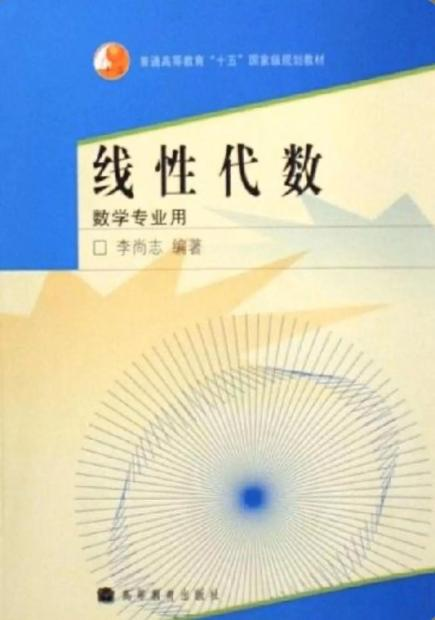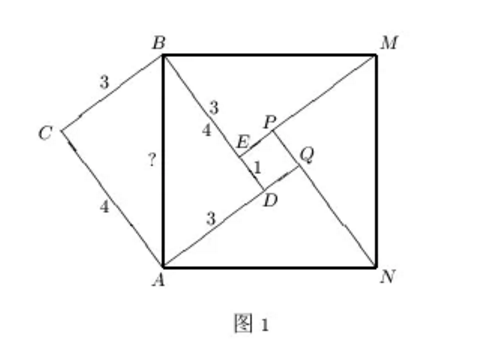## 【李尚志】为什么需要字母运算

【老万按】李尚志老师是中国自己培养的第一批博士之一。他曾任中国科技大学数学系教授和系主任、北京航空航天大学理学院院长和数学与系统科学学院院长。这篇文章来自李老师正在撰写的新书，由他授权“老万故事会”首发，希望对中学生朋友们有所帮助。

～～～～～～～～～～～～～～～～～

【李老师前言】我在2003年上半年在科大防非典期间完成了我的《线性代数（数学专业）》的主要部分。该教材早就开始写，因为上课、开会、出差较多，写书的工作进展迟缓。非典期间不上课不开会不出差，终于有了快速进展，2005年完成书稿交给高教出版社，2006年5月出版。～～～～～～～～～～～～～～～～～

1000000 × 999998 = 999998000000，

(999999 + 1)(999999 - 1)，

999999 变成 999999 + 1，是原来的 1000000/999999 倍。要使乘积不变，另一个 999999 就应该乘 1000000/999999 的倒数 999999/1000000。

999999/1000000 = 1 - 1/1000000，
999998/999999  = 1 - 1/999999 及
1/1000000 < 1/999999
999999/1000000 > 999998/999999。

“具有相反意义的量”的基本含义是两个量可以相互抵消。到底怎么抵消，依赖于“相反意义”是什么意义：做加法相互抵消的量是相反数，做乘法相互抵消就是倒数。

“相反意义的量” 的“意义”不明确，不是一个数学名词。有一个数学名词“逆”是它的同义词。但“逆”也要区分“加法逆”、“乘法逆”。

a 的加法逆 b 满足 a + b = 0，b = -a 是 a 的相反数。
a 的乘法逆 c 满足 ac = 1，c = 1/a 是 a 的倒数。

(999999 + 1)(999999 - 1)

(999999 + 1)(999999 - 1)
= 999999(999999 - 1) + 1(999999 - 1)   （分配律）
= (999999 × 999999 - 999999) + (999999 - 1)  （分配律）
= 999999² + (-999999 + 999999) - 1
= 9999999² - 1  （加法结合律）

999999²
= (999999 + 1)(999999 - 1) + 1
= 999998000001。

999999² = 1000000 × 999998 + 1

(a + b)(a - b) = a(a - b) + b(a - b)  （分配律）
= [aa + a(-b)] + [ba + b(-b)]  （分配律）
= a² + [a(-b) + ba] + b(-b)  （加法结合律）
= a² + [-ab + ba] - b²
= a² + 0 - b²   （乘法交换律）
= a² - b²

(a + b)(a - b) = a² - b²

(a + b)(a - b) = a² - b²

(85 + 5)(85 - 5) = 85² - 5²
85²
= (85 + 5)(85 - 5) + 5²
= 90 × 80 + 25
= 7225。

100a 是整数 a 的 100 倍。不论 a 是多少，100a 的末两位都是 00。因此，100a + 2020 - b 的末两位就是 2020 - b 的末两位，与 a 没有关系。

2020 就是今年，b 是你的出生年。不论你谁，用 2020 减去出生年份 b 得到的 2020 - b 都是你今年的年龄。只要你没满 100 岁，年龄 2020 - b 就是两位数。

100a + (2020 - b) 的末两位与 2020 - b 相同，等于你的年龄。

⬜ + ⬜  =  8
+       +
⬜ -  ⬜  =  6
||       ||
13      8

1  +  7  =  8
+     +
12  -  1  =  6
||     ||
13     8

x   + (8 - x) =  8
+           +
(13 - x) -  x     =  6
||          ||
13         8

(13 - x) - x = 6

(13 - x) - x
= [13 + (-x)] + (-x)
= 13 + [(-x) + (-x)]
= 13 - 2x

3.5 + 4.5 = 8
+        +
9.5 -  3.5 = 6
||         ||
13        8

999999² = 1000000 × 999998 + 14 个三角形面积都等于 SABD = 6，总面积为 6 × 4 = 24cm² 。

4 个三角形面积都等于 ab/2，总面积为 (ab/2)×4 = 2ab。

DE²
= (b - a)²
= (b - a)(b - a)
= b(b - a) - a(b - a)
= bb - ba - ab + (-a)(-a)
= b² - ab -ab + a²
= b² - 2ab + a²

41 = AB² = (6 + x)²。

41
= (6+x)²
= 6² + 2×6x + x²
= 36 + 12x + x²
= 36 + x(12 + x)

x
= 5÷(12 + x)
= 5÷12.…
≈ 5÷12
≈ 0.4

AB ≈ 6.4。验算得 6.4² = 40.96 确实很接近 41，可知对角线 AB ≈ 6.4。

25 分解为大小不同的两个正整数的乘积，只能 25 = 25×1。

(a，b，c) = (p，(p² - 1)/2，(p² + 1)/2) 及
(2a，2b，2c) = (2p，p² - 1，p² + 1)。